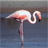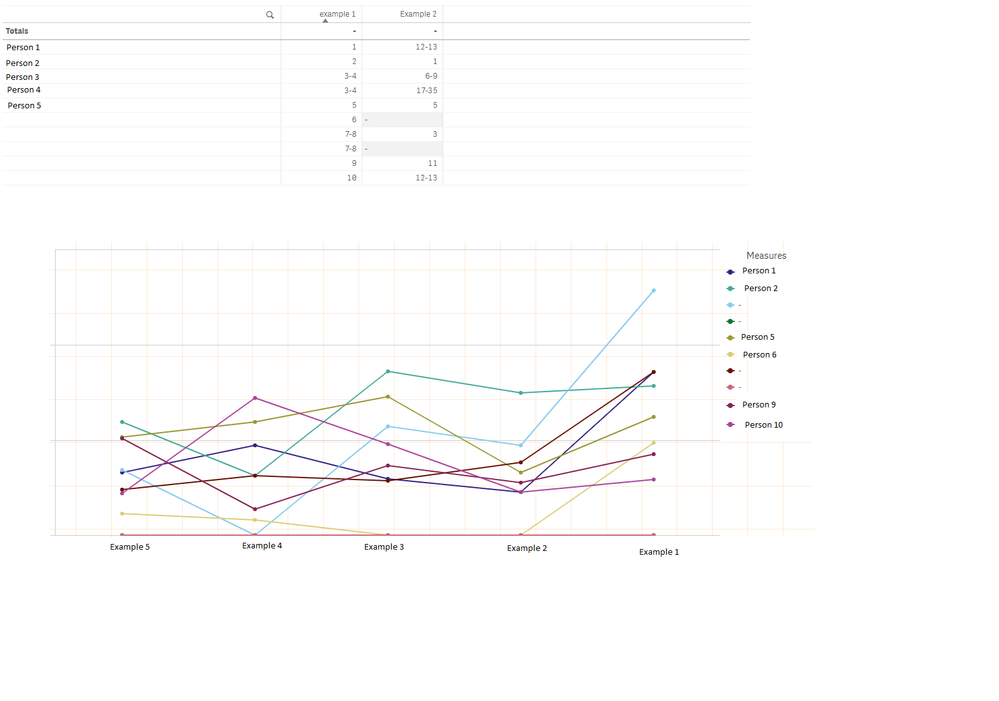# New to Qlik Sense

If you’re new to Qlik Sense, start with this Discussion Board and get up-to-speed quickly.

Announcements
Our next Qlik Insider session will cover new key capabilities. Join us August 11th REGISTER TODAY
cancel
Showing results for
Did you mean:Contributor II

## Tied ranks in measures

Hi,

I have a table that ranks each person's performance in each year (each example represents a year).

When I turn this into a line chart, the tied ranks aren't displayed. I know this is because my measures contain 'Rank ()=3' , Rank () =4 etc. but I can't figure out what to replace it with.

My measure for the 3rd person is:

aggr(sum( {<Response_%>} {<Question_% = {'Q1'} , Example_% = {'E1','E2','E3','E4','E5'},
[Response_%] = {"=rank(SUM({<Question_% = {'Q1'} , Response_% =- {'Other','No answer'} , Example_% = {'E1'}>} [Weighting_%]))=3"} >} [Weighting_%]) , Example_% )

/

aggr(sum({<Question_% = {'Q1'}, [Response_%] -= {'No answer'} >}[Weighting_%]), Example_% )Thanks

Labels (3)

• ### TiedRanks

0 RepliesTags
Community Browser